Next: October 11 Up: October 9 Previous: But first, structs and

Graph definitions

The intuitive notion of a graph is a drawing of (possibly labeled) nodes (often circles) with edges connecting some of them. Graphs turn out to be a model that many problems can be translated to, and often solved. You've already seen a special case of a graph, since a tree is an acyclic, connected graph with one node distinguished as the root (see definitions below).

Here are some formal definitions:

• A graph (denoted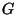) is a pair of sets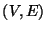, where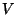is the set of vertices (or nodes) and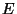is the set of edges. Vertices are usually the elements of the problem we're interested in. Each edge connects two vertices, so it can be represented as a pair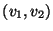.
• We can also denote the vertices of a graphas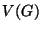and its edges as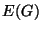. The number of vertices,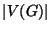is the called's order, and the number of edges,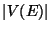is called's size.
• Since edges are ordered pairs,indicates there is an edge from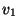to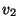, but not necessarily fromto-- if so there must be an edge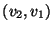in. When this distinction is important, we have a directed graph. In this course the edge relationship is usually symmetrical: there is an edgeif and only if there is an edge. In this case the edge is in fact the set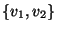(since sets have no order), and we have an undirected graph. A vertex may not have an edge to itself (unless we have a pseudograph).
• When two vertices share an edge they are adjacent'' (or neighbours''). The number of neighbours vertex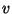has is called the degree of(for a directed graph we have in-degree and out-degree). A path is a list of vertices where each pair of vertices that are adjacent in the list are also adjacent in the graph.
• A circuit is a path that begins and ends on the same vertex without repeating any edges. A cycle is a path that begins and ends on the same vertex and doesn't repeat any vertices (and usually includes at least three vertices).
• Verticesandare connected if there is a path beginning withand ending with. Graphis connected if every pair of vertices inis connected.
• Graphis complete if every vertex is a neighbour of every other vertex. Clearly a complete graph is also connected. Ifis a complete, undirected graph, how many edges does it have? It turns out that two complete graphs of the same order (same number of vertices) are equivalent (isomorphic, which is defined later), so it makes sense to talk about the complete graph of order 2,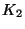, or order 4,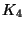, etc.
• A colouring is an assignment of colours to a graph so that
1. every vertex is assigned a colour.
2. no two neighbouring vertices are assigned the same colour
Usually we represent the colours by small integers. If it is possible to colour a graph with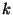colours, we say the graph iscolourable.'' If you know that it's possible with colour a graph withdifferent colours, then you can certainly colour it with more thancolours: beingcolourable doesn't require that allcolours are used, only thatcolours are enough.
• The chromatic number of graph, denoted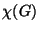, (that's the greek letter chi) is the minimum number of colours required to colour. It's easy to come up with an upper bound for, since youis certainlycolourable if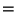. Usuallyis less than, but in the case of a complete graph,.
• To prove thatfor a given graph, you need to prove both thatis not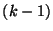colourable, and thatiscolourable.Next: October 11 Up: October 9 Previous: But first, structs and
Danny Heap 2002-12-16